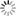# Episode List

OR

### Season 1

20 Sep. 1964
Don't Make Waves
7.5 (6)Lt. Anne Morgan arrives on Ranakai with her fellow Waves as replacements for the motor pool, and Commander Roger Adrian is not happy.

27 Sep. 1964
The Non-Permanent WaveBe the first one to add a plot.

4 Oct. 1964
Kill 'Em with KindnessBe the first one to add a plot.

11 Oct. 1964
Annie Shoots Down the CupidBe the first one to add a plot.

25 Oct. 1964
Lt. Morgan's Secret MarriageBe the first one to add a plot.

1 Nov. 1964
The Great Lipstick WarBe the first one to add a plot.

8 Nov. 1964Commander Adrian has another idea to get the Waves off the island by claiming Japanese forces are attacking. Lt. Morgan overhears the men and develops her own plan. Two different groups of fake enemy soldiers are soon facing off.

15 Nov. 1964
The Morale of Molly McGuireBe the first one to add a plot.

22 Nov. 1964Be the first one to add a plot.

29 Nov. 1964
Anne Bugs the EnemyBe the first one to add a plot.

6 Dec. 1964
Ranakai Flips Its WigBe the first one to add a plot.

13 Dec. 1964
My Son, the EggBe the first one to add a plot.

20 Dec. 1964
Selma, the Love TrapBe the first one to add a plot.

27 Dec. 1964
The Obstacle CourseBe the first one to add a plot.

3 Jan. 1965
Behind the Eight BallRoberta's old show biz friend Alex arrives, giving the Waves an idea on how Roberta can pass her ratings test. Alex will hypnotize her but in a demonstration Beasley is changed into a confident ladies man. Commander Adrian falls under a similar spell.

10 Jan. 1965
The StowawavesBe the first one to add a plot.

17 Jan. 1965
The Wolfman ComethBe the first one to add a plot.

24 Jan. 1965
Taurus to Aries to ChanceBe the first one to add a plot.

31 Jan. 1965Be the first one to add a plot.

7 Feb. 1965
The Arrival of Stanley StubbsBe the first one to add a plot.

14 Feb. 1965Be the first one to add a plot.

21 Feb. 1965
Three WishesBe the first one to add a plot.

28 Feb. 1965
Lieutenant Love, SirBe the first one to add a plot.

7 Mar. 1965Be the first one to add a plot.

14 Mar. 1965Be the first one to add a plot.

21 Mar. 1965
Filet on the HoofBe the first one to add a plot.

28 Mar. 1965
The Great Ranakai MutinyBe the first one to add a plot.

4 Apr. 1965
Anne Morgan's Home MoviesBe the first one to add a plot.

11 Apr. 1965
Witchcraft a Go-GoBe the first one to add a plot.

18 Apr. 1965
Once Upon an IslandCmdr. Adrian, Lt. Trotter, Ens. Beasley and the WAVES are stranded on an enemy occupied island.

25 Apr. 1965
Miss RanakaiBe the first one to add a plot.

2 May 1965
Operation Clip-Joint